Students can go through AP Board 7th Class Maths Notes Chapter 8 Congruency of Triangles to understand and remember the concepts easily.

## AP State Board Syllabus 7th Class Maths Notes Chapter 8 Congruency of Triangles

→ Two figures are said to be identical if their shapes are same.
Eg: Any two squares, circles or equilateral triangles.

→ Two figures are said to be congruent if they are identical in shape and equal in size.

→ Two line segments are congruent if they have same lengths.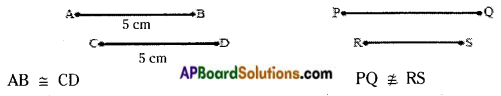→ Two triangles are congruent if the corresponding angles are equal.

→ We establish the congruency of the triangles by following criteria.

→ S.S.S. criterion: If three sides of a triangle are equal to the corresponding three sides of another triangle, then the triangles are congruent.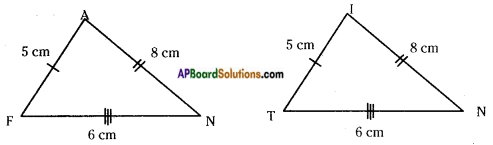FA = TI; AN = IN; FN = TN then △FAN ≅ △TIN

→ S.A.S. criterion: If two sides and the angle included between the two sides of a triangle are equal to the corresponding two sides and the included angle of another triangle, then the two triangles are congruent.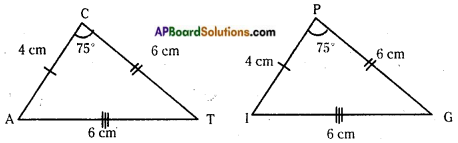CA = PI; ∠C = ∠P; CT = PG
then, △CAT ≅ △PIG

→ A.S.A. criterion : If two angles and the included side of a triangle are equal to the corresponding two angles and included side of another triangle then the triangles are congruent.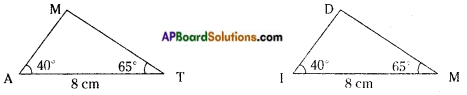∠A = ∠I; AT = IM; ∠T = ∠M then △MAT ≅ △DIM.→ R.H.S. criterion: In two right angled triangles, if the hypotenuse and one corresponding side are equal then they are congruent.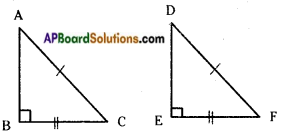∠B = ∠E = 90°
BC = EF
AC = DF
then △ABC ≅ △DEF

→ If by any criterion two triangles are congruent then all the corresponding parts are equal.